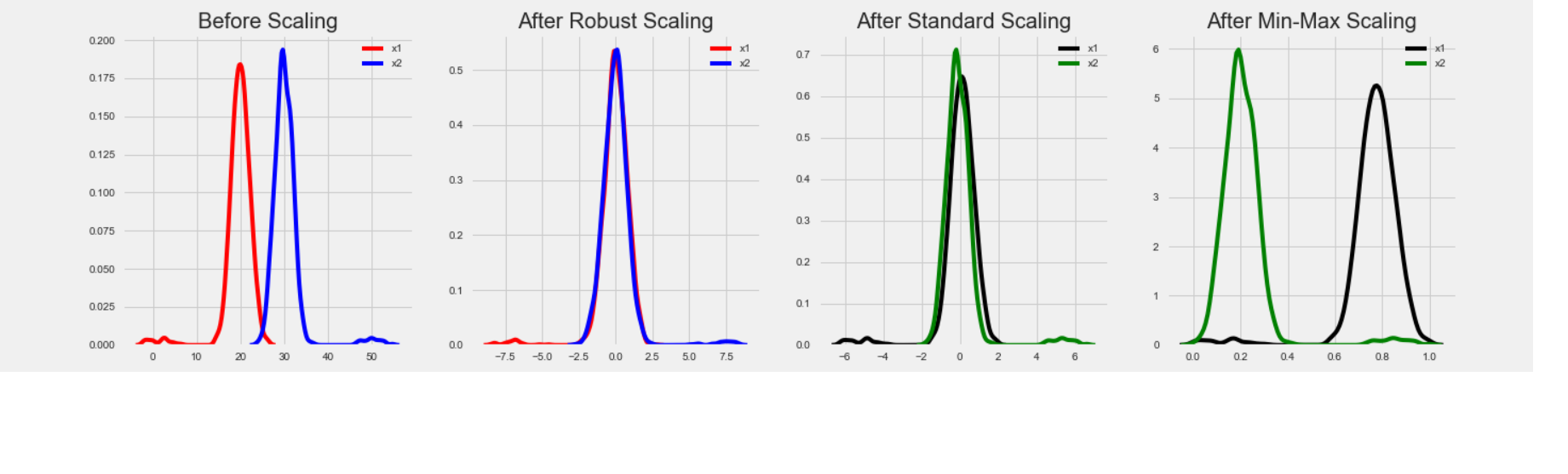Related Articles

# StandardScaler, MinMaxScaler and RobustScaler techniques – ML

• Difficulty Level : Medium
• Last Updated : 16 Jul, 2020

StandardScaler follows Standard Normal Distribution (SND). Therefore, it makes mean = 0 and scales the data to unit variance.
MinMaxScaler scales all the data features in the range [0, 1] or else in the range [-1, 1] if there are negative values in the dataset. This scaling compresses all the inliers in the narrow range [0, 0.005].
In the presence of outliers, StandardScaler does not guarantee balanced feature scales, due to the influence of the outliers while computing the empirical mean and standard deviation. This leads to the shrinkage in the range of the feature values.

By using RobustScaler(), we can remove the outliers and then use either StandardScaler or MinMaxScaler for preprocessing the dataset.

How RobustScaler works:
`classsklearn.preprocessing.RobustScaler(with_centering=True,with_scaling=True,quantile_range=(25.0, 75.0),copy=True,)`
It scales features using statistics that are robust to outliers. This method removes the median and scales the data in the range between 1st quartile and 3rd quartile. i.e., in between 25th quantile and 75th quantile range. This range is also called an Interquartile range.

The median and the interquartile range are then stored so that it could be used upon future data using the transform method. If outliers are present in the dataset, then the median and the interquartile range provide better results and outperform the sample mean and variance.

RobustScaler uses the interquartile range so that it is robust to outliers. Therefore its formula is as follows:Code: comparison between StandardScaler, MinMaxScaler and RobustScaler.

 `# Importing libraries``import` `pandas as pd``import` `numpy as np``from` `sklearn ``import` `preprocessing``import` `matplotlib``import` `matplotlib.pyplot as plt``import` `seaborn as sns ``%` `matplotlib inline``matplotlib.style.use(``'fivethirtyeight'``)`` ` `# data``x ``=` `pd.DataFrame({``    ``# Distribution with lower outliers``    ``'x1'``: np.concatenate([np.random.normal(``20``, ``2``, ``1000``), np.random.normal(``1``, ``2``, ``25``)]),``    ``# Distribution with higher outliers``    ``'x2'``: np.concatenate([np.random.normal(``30``, ``2``, ``1000``), np.random.normal(``50``, ``2``, ``25``)]),``})``np.random.normal`` ` `scaler ``=` `preprocessing.RobustScaler()``robust_df ``=` `scaler.fit_transform(x)``robust_df ``=` `pd.DataFrame(robust_df, columns ``=``[``'x1'``, ``'x2'``])`` ` `scaler ``=` `preprocessing.StandardScaler()``standard_df ``=` `scaler.fit_transform(x)``standard_df ``=` `pd.DataFrame(standard_df, columns ``=``[``'x1'``, ``'x2'``])`` ` `scaler ``=` `preprocessing.MinMaxScaler()``minmax_df ``=` `scaler.fit_transform(x)``minmax_df ``=` `pd.DataFrame(minmax_df, columns ``=``[``'x1'``, ``'x2'``])`` ` `fig, (ax1, ax2, ax3, ax4) ``=` `plt.subplots(ncols ``=` `4``, figsize ``=``(``20``, ``5``))``ax1.set_title(``'Before Scaling'``)`` ` `sns.kdeplot(x[``'x1'``], ax ``=` `ax1, color ``=``'r'``)``sns.kdeplot(x[``'x2'``], ax ``=` `ax1, color ``=``'b'``)``ax2.set_title(``'After Robust Scaling'``)`` ` `sns.kdeplot(robust_scaled_df[``'x1'``], ax ``=` `ax2, color ``=``'red'``)``sns.kdeplot(robust_scaled_df[``'x2'``], ax ``=` `ax2, color ``=``'blue'``)``ax3.set_title(``'After Standard Scaling'``)`` ` `sns.kdeplot(standard_df[``'x1'``], ax ``=` `ax3, color ``=``'black'``)``sns.kdeplot(standard_df[``'x2'``], ax ``=` `ax3, color ``=``'g'``)``ax4.set_title(``'After Min-Max Scaling'``)`` ` `sns.kdeplot(minmax_df[``'x1'``], ax ``=` `ax4, color ``=``'black'``)``sns.kdeplot(minmax_df[``'x2'``], ax ``=` `ax4, color ``=``'g'``)``plt.show()`

Output:Parameters of RobustScaler:

• with_centering: boolean: It is True by default. If the value is True, the data is centred before scaling. When it is applied on sparse matrices, the transform will raise an exception because centring them requires building a dense matrix, which generally is too large to fit in the memory.
• with_scaling: boolean: It is also set to True by default. It scales the data to the interquartile range.
• quantile_range: tuple(q_min, q_max), 0.0 < q_min < q_max < 100.0 : Quantile range is used to calculate scale. By default, it is set as below. Default: (25.0, 75.0) = (1st quantile, 3rd quantile) = IQR.
• copy: boolean It is an optional parameter. By default, it is True. If the input is already a NumPy array or a scipy.sparse CSC matrix and if axis = 1, avoid a copy by setting this parameter to False and instead perform inplace row normalization.

Attributes:

• center_: array of floats: The median value for each feature in the training set.
• scale_: array of floats: The scaled interquartile range for the feature in the training set.

Attention reader! Don’t stop learning now. Get hold of all the important Machine Learning Concepts with the Machine Learning Foundation Course at a student-friendly price and become industry ready.

My Personal Notes arrow_drop_up